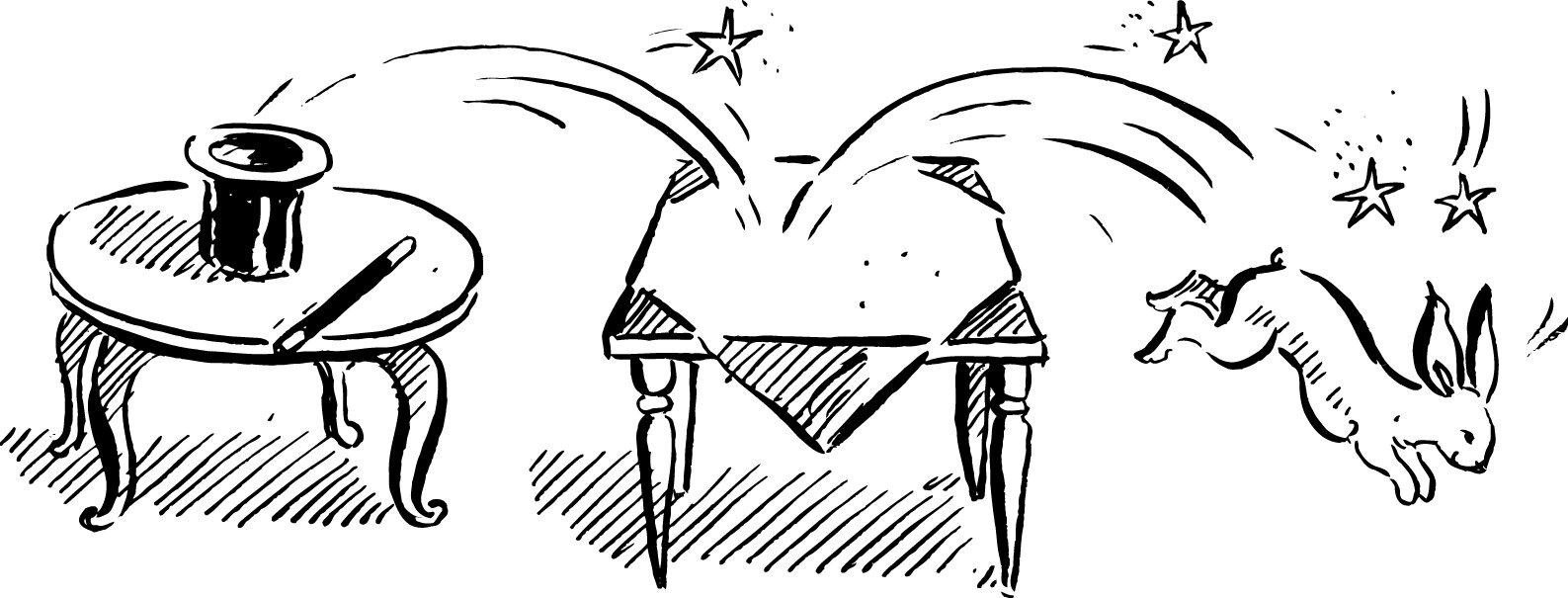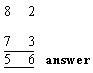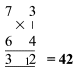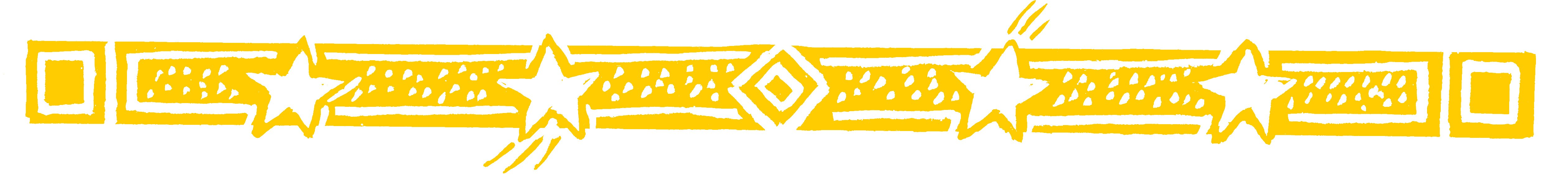TABLES MAGICDon’t know your tables?  Never mind, in this system you don’t need them beyond 5 × 5!

• Suppose you need 8 x 7

8 is 2 below 10 and 7 is 3 below 10.
Think of it like this:The answer is 56.
The diagram below shows how you get it.You subtract crosswise 8-3 or 7 - 2 to get 5,
the first figure of the answer.
And you multiply vertically: 2 x 3 to get 6,
the last figure of the answer.

That's all you do:

See how far the numbers are below 10, subtract one
number's deficiency from the other number, and
multiply the deficiencies together.

• 7 x 6 = 42Here there is a carry: the 1 in the 12 goes over to
make 3 into 4.

Question Comment !
 Tutorial Title Question Title No Questions 0 Questions Asked 0 Skipped Questions 0 Correctly Answered 0 Wrongly Answered 0 Total Question Attempts 0 Current Question Attempts 0

Skip Question

Reset Test

Halt Test

 No Questions 0 Questions Asked 0 Skipped Questions 0 Correctly Answered 0 Wrongly Answered 0 Total Question Attempts 0

Time Taken

CloseTables MagicThe whole heaven is number
and harmony
ARISTOTLE